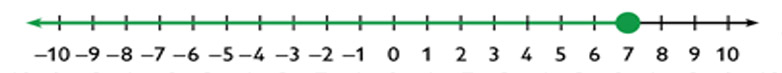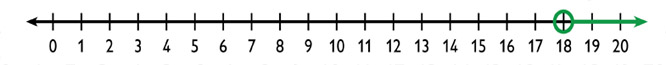Subject:
Algebra
Material Type:
Lesson Plan
Level:
Middle School
7
Provider:
Pearson
Tags:
7th Grade Mathematics, Equations, Graphing, Inequalities
Language:
English
Media Formats:
Text/HTML

# Self Check Exercise## Overview

Students work in pairs to critique and improve their work on the Self Check. Students complete a task similar to the Self Check with a partner.

# Key Concepts

To critique and improve the task from the Self Check and to complete a similar task with a partner, students use what they know about solving inequalities, graphing their solutions, and relating the inequalities to a real-world situation.

# Goals and Learning Objectives

• Solve algebraic inequalities.
• Graph the solutions of inequalities using number lines.
• Write word problems that match algebraic inequalities.
• Interpret the solution of an inequality in terms of a word problem.

# Critique

Students should look at the results of their Self Check task and the questions under the critique section.

SWD: Make sure all students have access to and can comprehend the information in the Intervention questions. Students with disabilities will benefit from explicit explanation of expectations. Use multiple means of representing the information from the Self Check questions (visual presentation of text, TTS, visual supports, etc.).

# Critique

Review your work on the Self Check problem and think about these questions:

• How can you check that your problem matches the inequality?
• How can you check that your solution to the inequality is correct?

# Lesson Guide

Discuss the Math Mission. Students will apply their understanding of algebraic inequalities to solve problems.

## Opening

Apply your understanding of algebraic inequalities to solve problems.

# Lesson Guide

Students work in pairs on revising their tasks from the Self Check

Support students as they work. Try not to make suggestions that move students to a particular approach. Instead ask questions that help students clarify their thinking.

# Interventions

Students do not understand when they need to reverse the direction of the inequality sign.

• Are you multiplying or dividing both sides of the inequality by a positive or negative number?
• What do you need to do when you multiply or divide both sides of an inequality by a negative number?

Neither student can think of a scenario that matches the inequality.

• Think about 66 as a score on a quiz.
• Think about 66 as the number of chairs in a restaurant.

Students' word problem does not match the inequality given.

• Work backward. Read the word problem you wrote.
• Write an inequality that represents it.
• Does it match the inequality you were given?

Students do not know how to connect the solution of the inequality to the solution to the word problem.

• Will all numbers on the solution graph make sense as solutions to your problem?1. $\begin{array}{c}8x+10\le 66\\ 8x+10-10\le 66-10\\ 8x\le 56\\ \frac{8x}{8}\le \frac{56}{8}\\ x\le 7\end{array}$

x is less than or equal to 7

2. Sample word problem: A bucket of balls weighs 66 ounces or less. If the bucket, without any balls, weighs 10 ounces and each ball weighs 8 ounces, how many balls could be in the bucket?
3. There could be any whole number of balls from 0 to 7.

## Work Time

Work with your partner to revise your work on the Self Check problem based on the previous questions and feedback from your partner.

Check that your solution is correct, and show the method you used to solve the inequality.

Self Check

1. Solve the inequality (show all your steps) and represent the solution on the given number line.

8x + 10 ≤ 66

2. Write a word problem that could be solved using the inequality.

# Lesson Guide

Students work in pairs on a task similar to the Self Check.

# Mathematical Practices

Mathematical Practice 1: Make sense of problems and persevere in solving them.

Students must make sense of the task. They must plan and carry out a solution strategy. After they complete the task, they need to go back and check that their solution makes sense. Students also have the opportunity to work with another student and try to understand his or her approach to the task.

Mathematical Practice 6: Attend to precision.

Students are asked to present their work in a clear, precise way. They should try to use correct mathematical language and symbols and to carefully label their work so that it is easy to follow.

# Interventions

Students do not understand when they need to reverse the direction of the inequality sign.

• Are you multiplying or dividing both sides of the inequality by a positive or negative number?
• What do you need to do when you multiply or divide both sides of an inequality by a negative number?

Neither student can think of a scenario that matches the inequality.

• Think about 80 as a score on a quiz.
• Think about 80 as the number of chairs in a restaurant.

Students' word problem does not match the inequality given.

• Work backwards. Read the word problem you wrote.
• Write an inequality that represents it.
• Does it match the inequality you were given?

Students do not know how to connect the solution of the inequality to the solution to the word problem.

• Will all numbers on the solution graph make sense as solutions to your problem?

• x is greater than 18

$\begin{array}{c}4x+8-8>80-8\\ 4x>72\\ \frac{4x}{4}>\frac{72}{4}\\ x>18\end{array}$• Sample word problem: A restaurant wants to be able to seat more than 80 people. It will have one table that seats 8 and the other tables will seat 4. How many tables of 4 will they need to have?
• There must be more than 18 tables that seat 4 (so, at least 19 tables that seat 4).

## Work Time

Use what you learned from the Self Check problem to approach this similar task.

• Solve the inequality (show all your steps) and represent the solution on the given number line:

4x + 8 > 80

• Write a word problem that could be solved using the inequality.

HANDOUT: Solving Inequalities
INTERACTIVE: Graph an Inequality

# Preparing for Ways of Thinking

While students are working, note different approaches to share during the Ways of Thinking discussion:

If students find it difficult to get started, these questions might be helpful:

• How did you organize your work?
• What steps did you use to solve the inequality?
• Is your solution to the inequality correct?
• How did you check to make sure the problem matches the inequality?

If several pairs of students are struggling with the same issue, write a relevant question on the board. You might ask a student who has performed well on a particular part of the task to help a pair that is struggling.

• What questions were you asked for feedback?
• How could you and your partner work together to address one or more of those feedback questions?

SWD: Consider the prerequisite skills for this task. Students with disabilities may need direct instruction on and guided practice with the skills needed to complete this task.

# Prepare a Presentation

• Explain the process you used to revise your work.
• Describe how you applied what you learned from the Self Check problem to solve a similar problem.

# Mathematics

Ask pairs of students who used different solution methods to present their work. Include students who made errors. Try to allow as many students as possible to share their word problems.

Have students make observations and ask questions as different pairs present their work. Encourage students to talk about how they revised their work based on feedback.

Ask questions such as the following:

• Did you reverse the direction of the inequality sign? Why or why not?
• Did you use a closed circle or an open circle on your number line? Why?
• How do you know that your number line shows all the solutions of the inequality?
• Are all the numbers graphed on the number line solutions to the problem? Why or why not?

# Ways of Thinking: Make Connections

Take notes your classmates’ approaches to revising the Self Check problem and applying what they learned to solve a similar problem.

## Hint:

• Why didn't you reverse the direction of the inequality sign?
• Why did you use a closed circle on your number line?
• How do you know that your number line shows all the solutions to the inequality?
• Are all the numbers on the number line solutions to the problem? Why or why not?